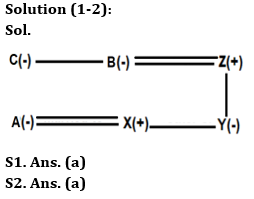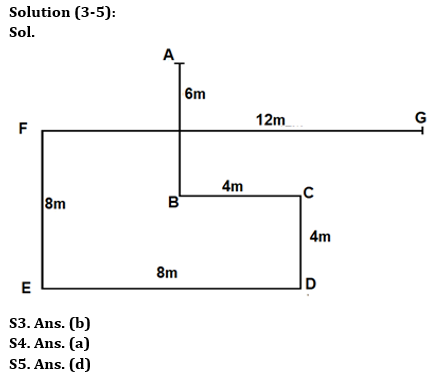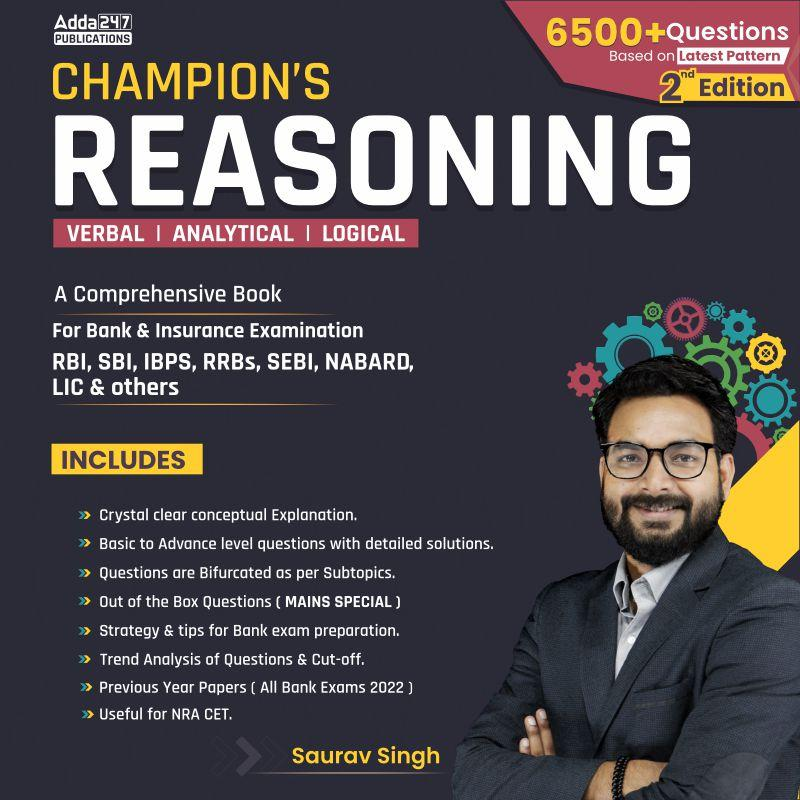Latest Banking jobs   »   IBPS reasoning

# Reasoning Quiz For IBPS PO Prelims 2023 -19th August

Directions (1-2): Answer the questions based on the information given below.
A family consists of six members A, B, X, Z, C and Y. There is no single parent in the family. There are only two generations in the family. Y is the only daughter of Z. B is the mother of X. C is the maternal aunt of X. A is the daughter-in-law of Z.

Q1. How is X related to Z?
(a) Son
(b) Daughter
(c) Brother
(d) Sister
(e) Can’t be determined

Q2. How will be the husband of B related to C?
(a) Brother-in-law
(b) Son-in-law
(c) Father-in-law
(d) Father
(e) None of these

Directions (3-5): Answer the questions based on the information given below.
A person starts walking in south direction from point A. After walking 6m, he reached at point B. From point B, he takes a left turn and walks for 4m to reach point C. Then, he turns right and walks for 4m towards point D. Now, he again turns right from point D and walks for 8m to reach point E. He turns right from point E and walks for 8m till point F. Finally, he again turns right from point F and walks for 12m till point G.

Q3. In which direction is Point A with respect to Point G?
(a) North-East
(b) North-west
(c) North
(d) West
(e) None of these

Q4. What is the shortest distance between point C and point G?
(a) √32m
(b) √38m
(c) √36m
(d) √40m
(e) None of these

Q5. In which direction is Point E with respect to Point A?
(a) North-East
(b) North-west
(c) North
(d) South-west
(e) None of these

Directions (6-10): Study the given arrangement of numbers, symbols & alphabets and answer the questions based on it.

1 A B 2 C D 4 ! F G H I J K L M N ^ O 6 E 5 \$ & P Q 7 8 % 9 R S T # U * V

Q6. How many even numbers are in between the element which is 6th from left and element which is 11th from right end?
(a) One
(b) Two
(c) Three
(d) Four
(e) None of the above

Q7. If we remove all the vowels from the given arrangement then which is exactly in the middle of ! and 6 in new arrangement?
(a) J
(b) H
(c) K
(d) L
(e) None of the above

Q8. If all the symbols are dropped, then which element will be eighth to the right of eighth element from left end?
(a) N
(b) M
(c) O
(d) 6
(e) None of the above

Q9. How many symbols are in between 2nd number from left end and 2nd number from right end?
(a) 2
(b) 3
(c) 4
(d) 5
(e) None of the above

Q10. If all the numbers are dropped, then which element will be 2nd to the left of 19th element from left end?
(a) M
(b) E
(c) O
(d) ^
(e) None of the above

SolutionsSolution (6-10):
S6. Ans. (b)
Sol. There are only two even numbers (4 and 6) between the element which is 6th from left end and 11th from right end.

S7. Ans. (c)
Sol. After removing all the vowels, we get K is exactly middle element between ! and 6.

S8. Ans. (a)
Sol. After dropping symbols: 1 A B 2 C D 4 F G H I J K L M N O 6 E 5 P Q 7 8 9 R S T U V
So, N is eighth to the right of eighth element from left end.

S9. Ans. (c)
Sol. There are 4 symbols (!, ^, \$, &) between 2nd number from left end and 2nd number from right end.

S10. Ans. (b)
Sol. After dropping numbers: A B C D ! F G H I J K L M N ^ O E \$ & P Q % R S T # U * V
So, E is second to the left of 19th element from left end.## FAQs

### When will the IBPS PO prelims 2023 be conducted?

IBPS PO Prelims will be conducted on 23, 30 September, and 1 October 2023.

#### Congratulations!Union Budget 2023-24: Free PDF